# Differentiation with Graphs

GCSELevel 8-9Cambridge iGCSEEdexcel iGCSE

## Differentiation with Graphs

Differentiation allows us to find the variable rate of change, so when looking at a graph, differentiation gives us the gradient at any given point.

Make sure you are happy with the following topic before continuing:

The following graph shows the line $y = 2x + 1$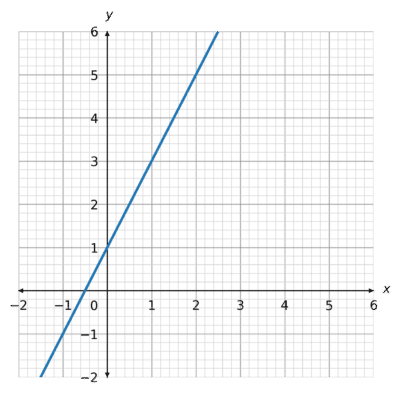We know to find the gradient we can find $2$ coordinates on the line and calculate $\dfrac{\text{change in} y}{\text{change in} x}$:

Using $(0,1)$ and $(3, 7)$:

$\dfrac{7-1}{3-0} = 2$

So, the gradient, or rate of change, of this line is $2$.

We could also calculate this by differentiating the equation of the line:

$y = 2x + 1\\$

$\dfrac{\text{d}y}{\text{d}x}=1\times 2x^{1-1} + 0 = 2$

Level 8-9GCSECambridge iGCSEEdexcel iGCSE

We can also use differentiation to find the gradients of curves at given points.

The following graph shows the curve $y = x^2 + 2$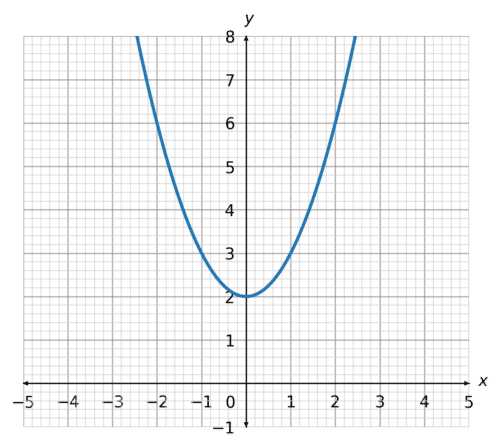Curves have many gradients, depending on which point you are looking at.

For example:

Find the gradient of the curve $y = x^2 + 2$ at the point $(2,6)$.

Differentiate:

$\dfrac{\text{d}y}{\text{d}x} = 2x$

Sub in the value of $x$ from $(2,6)$:

$\dfrac{\text{d}y}{\text{d}x} = 2(2) = 4$

Therefore, the gradient at the point $(2,6)$ is $4$.

If we were asked the same question but with the point $(4, 18)$, we would substitute $x=4$ into the derivative:

$\dfrac{\text{d}y}{\text{d}x} = 2(4) = 8$

Level 8-9GCSECambridge iGCSEEdexcel iGCSE

## Stationary Points

A stationary point on a curve occurs when the gradient is $0$.

The curve below has $2$ stationary points, one that is a maximum point, and one that is a minimum point.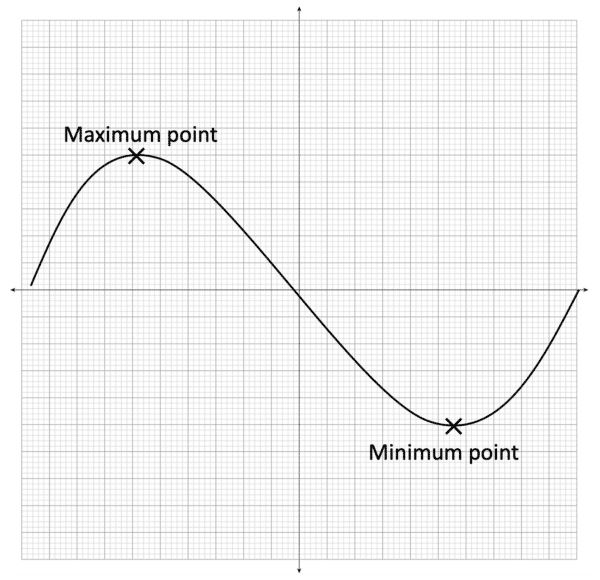The gradient at both the maximum and minimum point is $0$.

Finding the Coordinates of Stationary points:

For example, if a curve had the equation $y = 2x^2 + 4$, we can use differentiation to find the coordinates of the stationary point(s).

$\dfrac{\text{d}y}{\text{d}x} = 4x$

At a stationary point, we know the gradient, $4x$ is $0$:

$4x = 0\\$

We solve this equation:

$x = 0$

Therefore, the stationary point occurs when $x=0$. To find the full coordinate, we can sub this $x$ coordinate back into the curve’s equation:

$y = 2(0^2) + 4 = 4$

Coordinate: $(0,4)$

Level 8-9GCSECambridge iGCSEEdexcel iGCSE

## Example 1: Gradients of Lines

Find the gradients of the $3$ lines on this graph:

[3 marks]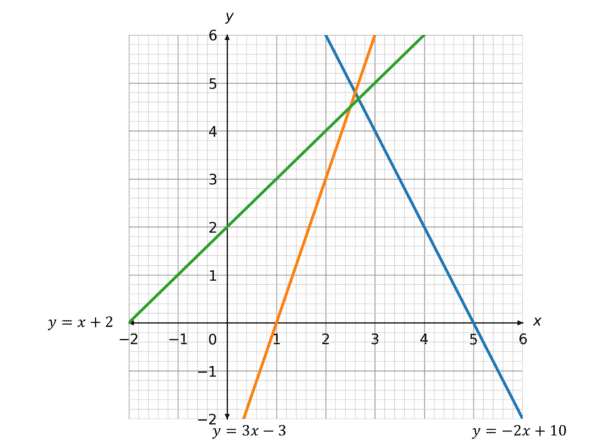$y = -2x + 10\\$ $\dfrac{\text{d}y}{\text{d}x} = -2$

$y = 3x - 3\\$ $\dfrac{\text{d}y}{\text{d}x} = 3$

$y = x + 2\\$

$\dfrac{\text{d}y}{\text{d}x} = 1$

Level 8-9GCSECambridge iGCSEEdexcel iGCSE

## Example 2: Stationary Points

Find the coordinates of the stationary point of the following curve, $y = 3x^2+12x+1$, deciding whether this is a minimum or maximum point.

[4 marks]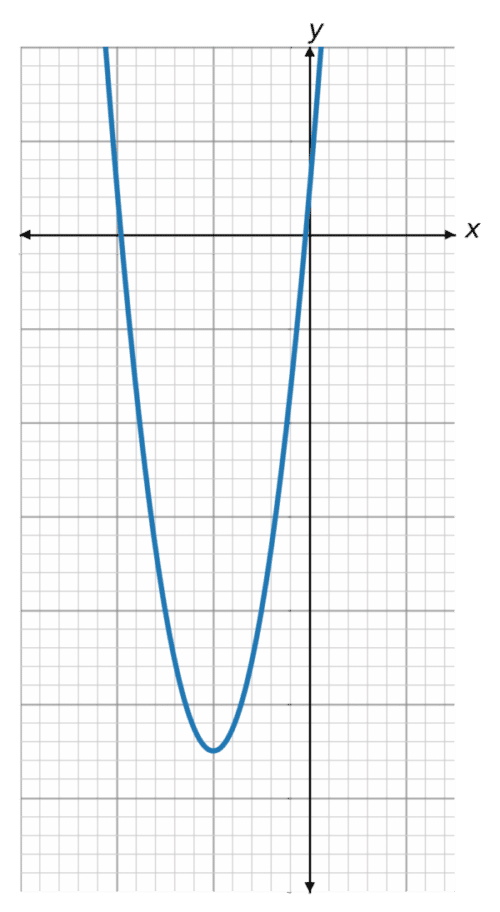We can differentiate to find the gradient:

$y=3x^2+12x+1\\$ $\dfrac{\text{d}y}{\text{d}x}=6x+12$

At the stationary point, the gradient will be $0$.

$\dfrac{\text{d}y}{\text{d}x}=6x+12=0\\$ $6x=-12\\$ $x=-2$

Sub this into the equation to find the $y$ coordinate:

$y=3(-2)^2+12(-2)+1\\$ $y=-11$

Coordinate: $(-2,11)$

We can see from the graph that this is a minimum point, as the curve decreases to this point, and then increases after.

Level 8-9GCSECambridge iGCSEEdexcel iGCSE

## Differentiation with Graphs Example Questions

Differentiate:

$\dfrac{\text{d}y}{\text{d}x}=4$

The gradient of the line is $4$.

Differentiate:

$\dfrac{\text{d}y}{\text{d}x}=-2x+4$

Stationary point occurs when the gradient is $0$:

$-2x+4=0\\$ $2x=4\\$ $x=2\\$

Sub $x$ into the curve equation:

$y=-(2)^2+4(2)+6$

$y =10$

$(2,10)$

This is a maximum point.

Differentiate:

$\dfrac{\text{d}y}{\text{d}x} = x^2+4x+3$

Gradient will be $0$ at the stationary points, so:

$x^2+4x+3=0\\$ $(x+3)(x+1)=0\\$

$x=-3$ and $x=-1$

When $x=-3$

$y=\dfrac{1}{3}(-3)^3+2(-3)^2+3(-3)+1 =1$

Stationary point: $(-3, 1)$

When $x=-1$

$y=\dfrac{1}{3}(-1)^3+2(-1)^2+3(-1)+1 =-\dfrac{1}{3}$

Stationary point: $\left(-1, -\dfrac{1}{3}\right)$

## You May Also Like...### MME Learning Portal

Online exams, practice questions and revision videos for every GCSE level 9-1 topic! No fees, no trial period, just totally free access to the UK’s best GCSE maths revision platform.

£0.00